# Class 10 Maths Extra Questions for Polynomials

Given below are the Class 10 Maths Extra questions for Polynomials with amswers. These are important questions for the examination
Questions are of below format
a. Match the column
b. Multiple choice questions
c. True and False
d. Factorize
e. Division
The questions are based on below concepts a. Finding Zero's Questions
c. Word Problems
d. Graph Questions

## Match the column

A.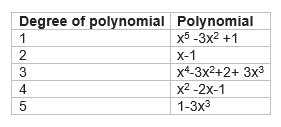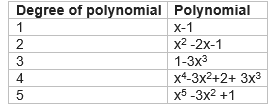B.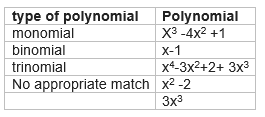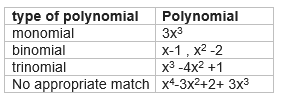C.
P(x)=5x3 -3x2+7x+2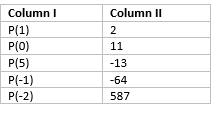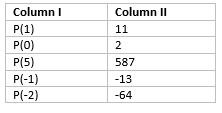D.
 Graph of polynomial Number of Zeros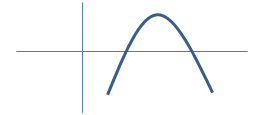012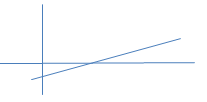3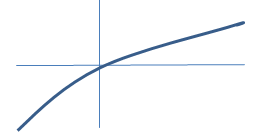4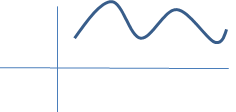5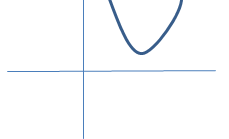6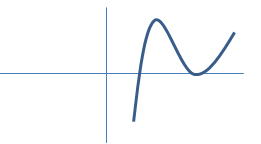7

a. it cuts the x-axis at two points ,so 2 zeroes
b. it cuts the x-axis at four points ,so 4 zeroes
c. Since it does not cut the axis, so  0 zeroes
d. it cuts the x-axis at 1 points ,so 1 zero’s
e. it cuts the x-axis at 1 points ,so 1 zero’s
f. Since it does not cut the axis, so  0 zeroes
g. Since it does not cut the axis, so  0 zeroes
h.   it cuts the x-axis at two points ,so 2 zeroes

E.
 Graph of polynomial Type of polynomial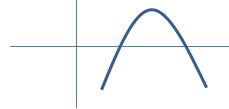Linear polynomialQuadratic polynomial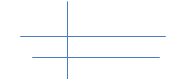Cubic polynomial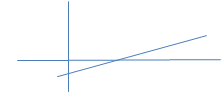Constant polynomial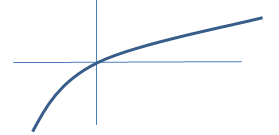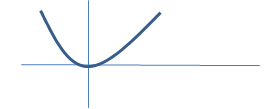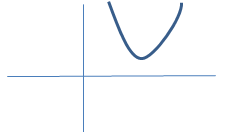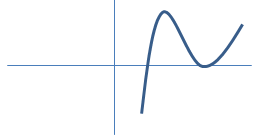b. Three zeroes,So cubic polynomial
c. Constant value polynomial
d. Linear polynomial
e. One zeroes but not straight line. So no appropriate match found
e. Cubic as has three zeroes ,two of them same

## Multiple choice Questions

Question 1
Find the remainder  when x4+x3-2x2+x+1 is divided by x-1
a.1
b.5
c.2
d. 3

Let P(x) =x4+x3-2x2+x+1
Remainder when divide by x-1
P(1) = 1+ 1-2+1+1=2

Question 2
Which of these identities is not true?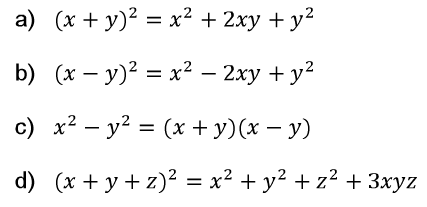Question 3
If a and b are the zeroes of the polynomial  x2-11x +30, Find the value of a3 + b3
a.134
b.412
c.256
d.341

a3 + b3= (a+b) (a2+b2-ab)=(a+b) {(a+b)2 -3ab}
Now a+b=-(-11)/1=11
ab=30
So a3+b3=11( 121 -90)=341

Question 4
S(x) = px2+(p-2)x +2. If  2 is the zero of this polynomial,what is the value of p
a.-1
b.1/2
c. -1/2
d. +1

S(2)=4p+0+2=0  => p=-1/2

Question 5
if the zeroes of the quadratic equation are 11 and 2 ,what is expression for quadratic
a. x2-13x+22
b. x2-11x+22
c. x2-13x-22
d. x2+13x-22

P(x) =(x-11)(x-2)

Question 6
p(x) = x4 -6x3 +16x2 -25x +10
q(x) = x2-2x+k
It is given
p(x) = r(x) q(x) + (x+a)
Find the value of k and a
a.2,-2
b. 5 ,-5
c. 7,3
d. 3,-1

Dividing p(x) by q(x) ,we get the remainder
(2k-9)x -(8-k)k +10
Comparing this with (x+a)
We get
K=5 and a=-5

Question 7
A cubic polynomial is given below
S(x) =x3 -3x2+x+1
The zeroes of the polynomial are given as (p-q) ,p  and (p+q). What is the value p and q
a. p=1,q=√2 or -√2
b. p=1,q=2 or -2
c. p=1,q=1 or -1
d. None of these

(a)

Question 8
If the zeroes of the quadratic polynomial ax2 + bx + c, c ≠ 0 are equal, then
a. c and a have opposite signs
b. c and b have opposite signs
c. c and a have the same sign
d. c and b have the same sign

Given that, the zeroes of the quadratic polynomial ax2 + bx + c , c ≠ 0 are equal
Value of discriminant (D) has to be zero
b2– 4ac = 0
b2 = 4ac
Since L.H.S. (b2) can never be negative
R.H.S. also can never be negative.
a and c must have same sign

Question 9
What are the zeroes of the Polynomial $p(x)=6x^2 - 7x -3$
a. (3/2) , (-1/3)
b. (3/2) , (1/3)
c. (-3/2) , (1/3)
d. (-3/2) , (-1/3)

$p(x)=6x^2 - 7x -3$
$p(x) = 6x^2 -9x + 2x -3 = 3x(2x -3) + 1(2x-3)=(3x+1)(2x-3) So roots are (3/2) , (-1/3) Hence option(a) is correct Question 10 What are the zeroes of the Polynomial$p(x)=x^2 + 7x +10$a. (2) , (-5) b. (-2) , (5) c. (2) , (5) d. (-2) , (-5) Answer ">$p(x)=x^2 + 7x +10p(x) = x^2 + 5x +2x +10 = x(x +5) + 2(x+5)=(x+2)(x+5)
So roots are (-2) , (-5)
Hence option(d) is correct

## True or False statement

1. P(x) =x-1 and g(x) =x2-2x +1 .  p(x) is a factor of g(x)
2. The factor of 3x2 -x-4 are  (x+1)(3x-4)
3. Every linear polynomial has only one zero
4. Every real number is the zero’s of zero polynomial
5. A binomial may have degree 6
6. 1,2 are the zeroes of x2-3x+2
7. The degree of zero polynomial is not defined
8.  Graph of polynomial (x2-1) meets the  x-axis at one point
9. Graph of constant polynomial never meets x axis

1. True, as g(1)=0
2. True, we can get this by split method
3. True
4. True
5. True , example x6 +1
6. True
7. True
8. False as it meets at two points
9.  True

## Factorize following

1. x2 +9x+18
2. 3x3 -x2-3x+1
3. x3-23x2+142x-120
4. 1+8x3

a.(x+6)(x+3)
b.(3x-1)(x-1)(x+1)
c.(x-1)(x-10)(x-12)
d. (2x+1)(4x2-2x+1)

## Division of polynomial

s(x) =r(x) s(x) + w(x)
Find the value of r(x) and w(x) in each case
a. p(x) =x4+x3+2x2+3x+4
s(x) =x+2
b. p(x) =x4+4
s(x)=x2+x+1

a. r(x)=x3-x2 +4x-5   w(x)=14
b. r(x)=x2-x  w(x) =x+4

Question 1
Find a quadratic polynomial whose zeroes are $5 + \sqrt {2}$ and $5 - \sqrt {2}$

If $\alpha$ and $\beta$ are the zeroes of the polynomials
$x^2 - (\alpha + \beta) + \alpha \beta$
Here $\alpha + \beta =5 + \sqrt {2} + 5 - \sqrt {2} =10$
$\alpha \beta =(5 + \sqrt {2})(5 - \sqrt {2}) = 25 -4 =21$
$x^2 - 10x + 21$

Question 2
If a and b are zeroes of quadratic polynomial $kx^2 + 4x + 4$, find the value of k such that $(a+b )^2- 2ab= 24$.

Here $a + b= -\frac {4}{k}$
$ab=\frac {4}{k}$
Now $(a+b )^2- 2ab= 24$
$(-\frac {4}{k})^2 -2 \frac {4}{k}=24$
$16-8k=24k^2$ or $3K^2 +k-2=0$
$k=-1$ or $k=\frac {2}{3}$

Question 3
If one zero of $3x^2 - 4x + p$ is reciprocal to the other, then find the value of p

Let a be one of zero, then 1/a be the another zero
Now $a \times \frac {1}{a} =\frac {p}{3}$
or p=3

Question 4
If p and q are the zeroes of $p(x) = kx^2 - 3x + 2k$ and $p+q=pq$ then find the value of k.

Here $p + q=\frac {3}{k}$
$pq=\frac {2k}{k}$
Now
$p+q=pq$
$\frac {3}{k} = 2$
$k= \frac {3}{2}$

Question 5
If a and b are zeroes of $x^2 - 6x + k$. What is the value of k, if $3a+2b= 20$?

Here $a + b= - \frac {-6}{1} = 6$ --(1)
$ab= k$ -(3)
Given $3a+2b= 20$ --(2)
Solving (1) and (2)
a=14 and b=-8
Now substituting these values in (3)
k=-112

Question 6
If one zero of the quadratic polynomial $x^2 + 3x + k$ is 2, then find the value of k.

Since 2 is zero of the quadratic polynomial
$2^2 + 3 \times 2 + k=0$
k=-10

Question 7
Find the zeroes of the quadratic polynomial $x^2 + 8x + 16$ and verify the relationship between the zeroes and the coefficients.

Now $x^2 + 8x + 16$
$= x^2 + 4x + 4x + 16 = x(x+4) + 4(x+ 4) = (x+4)^2$
So zeroes are -4 ,-4

Now if $k_1$ ,$k_2$ are the roots of quadratic polynomials $ax^2 + bx + c$, then relationship between them is given by
$k_1 + k_2 = -\frac {b}{a}$ and $k_1 k_2= \frac {c}{a}$

Now lets verify the relationship in the above polynomial
$-4 + (-4) = -\frac {8}{1}$
$-8=-8$

Also
$(-4) \times (-4) = \frac {16}{1}$
16=16

Question 8
Find the value of a and b, if they are the zeroes of polynomial x2 + ax + b.

Here
$a + b= -a$ or $2a + b=0$ -(1)
Also $ab= b$ or $a=1$
Substituting the value of a in (1)
b=-2
Hence a=1 and b=-2

Question 9
If m and n are the zeroes of the polynomial $3x^2 + 11x - 4$, find the value of $\frac {m}{n} + \frac {n}{m}$

Here $m + n= = \frac {11}{3}$
$mn= \frac {-4}{3}$
Now
$\frac {m}{n} + \frac {n}{m} = \frac {m^2 + n^2}{mn} = \frac {(m+n)^2 -2mn}{mn} = \frac {-145}{12}$

Question 10
Show that 2, -1 and 1/2 are the zeroes of the cubic polynomial
$p(x) = 2x^3 - 3x^2 - 3x + 2$

and then verify that the sum of the zeroes =-(Coeff of x2/Coeff of x3)

Question 11
Find the zeroes of the polynomial $f(x) = 4 \sqrt {3} x^2 + 5x - 2 \sqrt {3}$ , and verify the relationship between the zeroes and its coefficients.

$4 \sqrt {3} x^2 + 5x - 2 \sqrt {3}$
$= 4 \sqrt {3} x^2 + 8x -3x - 2 \sqrt {3}$
$= 4x (\sqrt {3}x + 2) - \sqrt {3}(\sqrt {3}x + 2)$
$= (4x - \sqrt {3})(\sqrt {3}x + 2)$

Question 12
If p and q are the zeroes of the polynomial $f(x) = x^2 - 5x + k$ such that $p-q= 1$, find the value of k.

Here $p + q = 5$ -(1)
$pq= k$ -(2)
Given $p-q= 1$ - (3)
From (1) and (3)
p=3, q=2
Substituting these values in (2)
k=6

Question 14
If a and b are the zeroes of the quadratic polynomial $f(x) = x^2 - 4x + 3$, find the value of $a^4 b^3 + a^3 b^4$.

Here $a + b=-\frac {-4}{1} =4$
$ab=3$
$a^4 b^3 + a^3 b^4 = a^3b^3(a +b) = 27 \times 4 = 108$

Question 15 If a and b are the zeroes of the quadratic polynomial $f(x) = x^2 - px + q$, prove that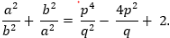Here
$a + b =p$ and $ab=q$
Now LHS
$= \frac {a^2}{b^2} + \frac {b^2}{a^2}$
$= (\frac {a}{b} + \frac {b}{a})^2 -2$
$= ( \frac {a^2 + b^2}{ab})^2 -2$
$=(\frac {(a+b)^2 - 2ab}{ab})^2 -2 = (\frac {p^2 -2q}{q})^2 -2$
$=\frac {p^4 +4q^2 -4p^2q}{q^2} -2 = \frac {p^4}{q^2} +4 - 4\frac {p^2}{q} -2$
$= \frac {p^4}{q^2} - 4\frac {p^2}{q} +2$
=RHS

Question 16
If two zeroes of the polynomial $f(x) =x^3 - 4x^2 - 3x + 12$ are √3 and -√3 then find its third zero.

If √3 and -√3 are zeroes of the polynomial, then $(x- \sqrt {3})(x+ \sqrt {3}) = x^2 -3$ should be factor of it.
$x^3 - 4x^2 - 3x + 12= x^2(x-4) -3(x -4) = (x^2 -3)(x-4)$
Clearly the other zero is 4

Question 17
If l and m are zeroes of the polynomial $p(x) = 2x^2 - 5x + 7$, find a polynomial whose zeroes are $2l+ 3$ and $2m+ 3$.

Here $l+m= \frac {5}{2}$
$lm= \frac {7}{2}$

Now the polynomial whose zeroes are $2l+ 3$ and $2m+ 3$ is given by
$x^2 - (2l+3 + 2m + 3)x +(2l+3)(2m+3)=x^2 -2(l+m)x -6x + 4lm + 6l + 6m +9$
$=x^2 -10x -6x + 14 + 6(l+m) +9$
$=x^2 -16x +14+15+9=x^2 -16x + 38$

Question 18
If p ,q and r are zeroes of polynomial $6x^3+ 3x^2 - 5x + 1$, then find the value of $\frac {1}{p} + \frac {1}{q} + \frac {1}{r}$.

Here $pq + qr+ pr=\frac {-5}{6}=- \frac {5}{6}$
$pqr= -\frac {1}{6}$
Now
$\frac {1}{p} + \frac {1}{q} + \frac {1}{r}$
$= \frac {pq+ qr + pr}{pqr} =5$

Question 19
If the zeroes of the polynomial $f(x) = ax^3 + 3bx^2 + 3cx + d$ are in A. P., prove that $2b^3 - 3abc + a^2d = 0$.

Given Roots of given polynomial is in AP
let p-q, p, and p+q are the roots of
$f( x) = ax^3 + 3bx^2 + 3cx + d$
Now we know that
$sum \; of \; roots = - \frac {coefficient \; of \; x^2} {coefficient \;of\; x^3}$
$p-q +p + p+q = - \frac {3b}{a}$
or $3p = - \frac {3b}{a}$
or $p = - \frac {b}{a}$ -----------(1)
Also,
$sum \; of \; products \; of \; two roots = \frac {coefficient \; of\; x}{coefficient \; of \; x^3}$
$(p -q)\times p + p \times (p+q) + (p-q)(p+q)=\frac {3c}{a}$
$3p^2 - q^2 = \frac {3c}{a}$ ------------------(2)

Also,
$products \; of \; all \; roots = -\frac {constant }{coefficient \; of \; x^3}$
$(p-q)\times p \times (p+q) = -\frac {d}{a}$
$p^3 - pq^2 = -\frac {d}{a}$ ----------(3)

Substituting the value of p from (1) in (2), we get the value of q as
$3 (-\frac {b}{a})^2 - q^2 = \frac {3c}{a}$
or
$q^2 = 3 \frac {b^2}{a^2} - 3 \frac {c}{a}$ ----------(4)

Now that we have got the values of p and q, substituting these in equation (3)

$(- \frac {b}{a})^3 +\frac {b}{a}( 3 \frac {b^2}{a^2} -3\frac {c}{a}) = -\frac {d}{a}$

$- \frac {b^3}{a^3} + \frac {b}{a}(3 \frac {b^2}{a^2} -3\frac {c}{a} ) = -\frac {d}{a}$

$- \frac {b^3}{a^3} +3 \frac {b^3}{a^3} -3 \frac {bc}{a^2} = -\frac {d}{a}$

$2\frac {b^3}{a^3} -3 \frac {bc}{a^2} = -\frac {d}{a}$

$2b^3 -3abc + a^2d = 0$

Question 20
Obtain all zeroes of the polynomial $f(x) = 2x^4 - 2x^3 - 7x^2 + 3x + 6$, if its two zeros are √3/2 and -√3/2

If √(3/2) and -√(3/2) are zeroes of the polynomial, then $(x- \sqrt {3/2})(x+ \sqrt {3/2}) = 2x^2 -3$ should be factor of it.
$f(x) = 2x^4 - 2x^3 - 7x^2 + 3x + 6$
Lets use division algorithm to find the other factors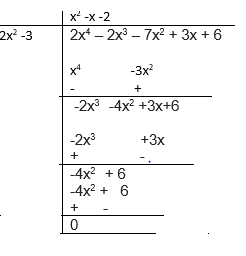So,
$2x^4 - 2x^3 - 7x^2 + 3x + 6= (2x^2 -3)(x^2 -x -2) = (2x^2 -3)(x^2 -2x + x-2) =(2x^2 -3)(x-2)(x+1)$
So other zeros are x=2 and x=-1

Question 21
The graphs of y = p(x) are given in below figure, for some polynomials p(x). Find the number of zeroes of p(x), in each case.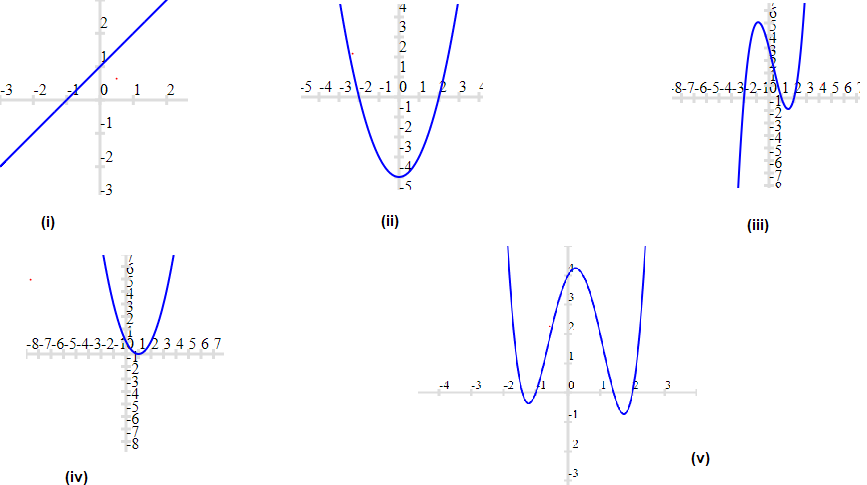i. 1
ii. 2
iii. 3
iv 1
v. 4

## Summary

This Class 10 Maths Extra Questions for Polynomials with answers is prepared keeping in mind the latest syllabus of CBSE . This has been designed in a way to improve the academic performance of the students. If you find mistakes , please do provide the feedback on the mail.Go back to Class 10 Main Page using below links

### Practice Question

Question 1 What is $1 - \sqrt {3}$ ?
A) Non terminating repeating
B) Non terminating non repeating
C) Terminating
D) None of the above
Question 2 The volume of the largest right circular cone that can be cut out from a cube of edge 4.2 cm is?
A) 19.4 cm3
B) 12 cm3
C) 78.6 cm3
D) 58.2 cm3
Question 3 The sum of the first three terms of an AP is 33. If the product of the first and the third term exceeds the second term by 29, the AP is ?
A) 2 ,21,11
B) 1,10,19
C) -1 ,8,17
D) 2 ,11,20